Courses

# Design Of Bolted Joints Mechanical Engineering Notes | EduRev

## Mechanical Engineering : Design Of Bolted Joints Mechanical Engineering Notes | EduRev

The document Design Of Bolted Joints Mechanical Engineering Notes | EduRev is a part of the Mechanical Engineering Course Machine Design.
All you need of Mechanical Engineering at this link: Mechanical Engineering

Stresses in screw fastenings

It is necessary to determine the stresses in screw fastening due to both static and dynamic loading in order to determine their dimensions. In order to design for static loading both initial tightening and external loadings need be known.

Initial tightening load

When a nut is tightened over a screw following stresses are induced:
(a) Tensile stresses due to stretching of the bolt
(b) Torsional shear stress due to frictional resistance at the threads.
(c) Shear stress across threads
(d) Compressive or crushing stress on the threads
(e) Bending stress if the surfaces under the bolt head or nut are not perfectly normal to the bolt axis.

(a) Tensile stress

Since none of the above mentioned stresses can be accurately determined bolts are usually designed on the basis of direct tensile stress with a large factor of safety. The initial tension in the bolt may be estimated by an empirical relation P1=284 d kN, where the nominal bolt diameter d is given in mm. The relation is used for making the joint leak proof. If leak proofing is not required half of the above estimated load may be used. However, since initial stress is inversely

proportional to square of the diameter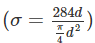bolts of smaller diameter such as M16 or M8 may fail during initial tightening. In such cases torque wrenches must be used to apply known load.

The torque in wrenches is given by T= C P1d where, C is a constant depending on coefficient of friction at the mating surfaces, P1 is tightening up load and d is the bolt diameter.

(b) Torsional shear stress This is given by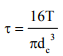where T is the torque and d the core diameter. We may relate torque T to the tightening load P1 in a power screw configuration (figure-4.4.1.1.1 ) and taking collar friction into account we may write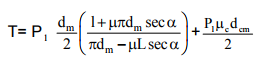where d and dcm are the mean thread diameter and mean collar diameter respectively, and m  μ and μc are the coefficients of thread and collar friction respectively α is the semi thread angle. If we consider that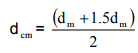then we may write T= C P1d m where C is a constant for a given arrangement. As discussed earlier similar equations are used to find the torque in a wrench.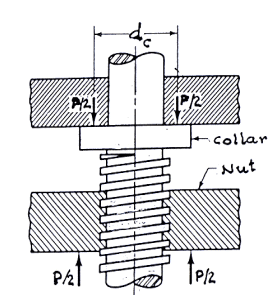(c) Shear stress across the threads This is given by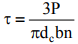where d is the core diameter and b is the base width of the thread and n is the number of threads sharing the load

(d) Crushing stress on threads This is given by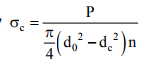where and d are the outside and core diameters as shown in figure- 4.4.1.1.1

(e) Bending stress If the underside of the bolt and the bolted part are not parallel as shown in figure4.4.1.1.2, the bolt may be subjected to bending and the bending stress may be given by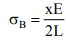where x is the difference in height between the extreme corners of the nut or bolt head, L is length of the bolt head shank and E is the young’s modulus.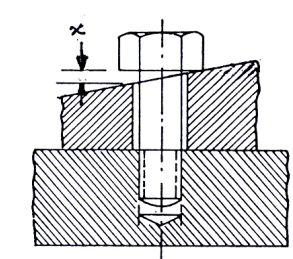4.4.1.1.2F- Development of bending stress in a bolt

Stresses due to an external load

If we consider an eye hook bolt as shown in figure- 4.4.1.2.1 where the complete machinery weight is supported by threaded portion of the bolt, then the bolt is subjected to an axial load and the weakest section will be at the root of the thread. On this basis we may write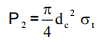where for fine threads dc =0.88d and for coarse threads dc =0.84d, d being the nominal diameter.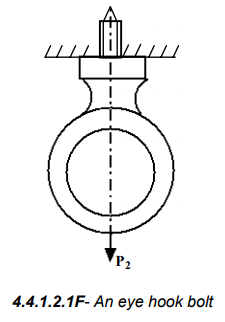Bolts are occasionally subjected to shear loads also, for example bolts in a flange coupling as shown in figure- 4.4.1.2.2. It should be remembered in design that shear stress on the bolts must be avoided as much as possible. However if this cannot be avoided the shear plane should be on the shank of the bolt and not the threaded portion. Bolt diameter in such cases may be found from the relation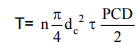where n is the number of bolts sharing the load, τ is the shear yield stress of the bolt material. If the bolt is subjected to both tensile and shear loads, the shank should be designed for shear and the threaded portion for tension. A diameter slightly larger than that required for both the cases should be used and it should be checked for failure using a suitable failure theory.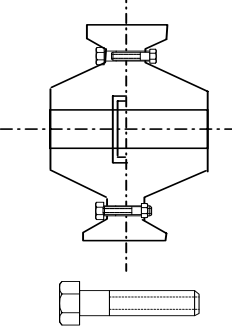4.4.1.2.2F- A typical rigid flange coupling

Combined effect of initial tightening load and external load

When a bolt is subjected to both initial tightening and external loads i.e. when a preloaded bolt is in tension or compression the resultant load on the bolt will depend on the relative elastic yielding of the bolt and the connected members. This situation may occur in steam engine cylinder cover joint for example. In this case the bolts are initially tightened and then the steam pressure applies a tensile load on the bolts. This is shown in figure-4.4.1.3.1 (a) and 4.4.1.3.1 (b).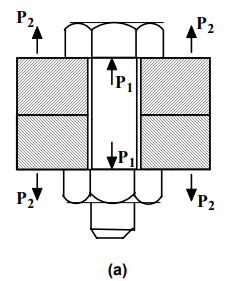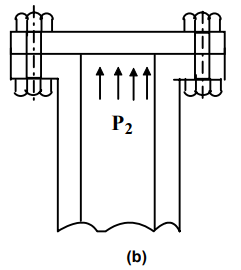4.4.1.3.1F- A bolted joint subjected to both initial tightening and external load

Initially due to preloading the bolt is elongated and the connected members are compressed. When the external load P is applied, the bolt deformation increases and the compression of the connected members decreases. Here P1 and P2 in figure 4.4.1.3.1 (a) are the tensile loads on the bolt due to initial tightening and external load respectively. The increase in bolt deformation is given by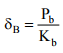and decrease in member compression is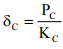where, Pb is the share of P2 in bolt, PC is the share of P2 in members, Kb  and Kc are the stiffnesses of bolt and members. If the parts are not separated then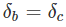and this gives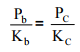Therefore, the total applied load P2 due to steam pressure is given by

P2= Pb+P

This gives Pb= P K, where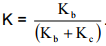. Therefore the resultant load on bolt is P1 +KP2 . Sometimes connected members may be more yielding than the bolt and this may occurs when a soft gasket is placed between the surfaces. Under these circumstances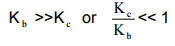and this gives K≈ 1. Therefore the total load P = P1 + P2

Normally K has a value around 0.25 or 0.5 for a hard copper gasket with long through bolts. On the other hand if Kc >>Kb , K approaches zero and the total load P equals the initial tightening load. This may occur when there is no soft gasket and metal to metal contact occurs. This is not desirable. Some typical values of the constant K are given in table 4.4.1.3.1.

 Type of joint K Metal to metal contact with through boltHard copper gasket with long through boltSoft copper gasket with through boltsSoft packing with through boltsSoft packing with studs 0-0.10.25-0.50.50- 0.750.75- 1.001.00

Leak proof joint

The above analysis is true as long as some initial compression exists. If the external load is large enough the compression will be completely removed and the whole external load will be carried by the bolt and the members may bodily separate leading to leakage. Therefore, the condition for leak proof joint is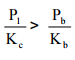Substituting Pb=P2 K and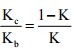the condition for a leak proof joint reduces to P1 >P2 (1-K). It is therefore necessary to maintain a minimum level of initial tightening to avoid leakage.

Joint separation

Clearly if the resultant load on a bolt vanishes a joint would separate and the condition for joint separating may be written as P1+KP2 =0

Therefore if P1 >KP 2 and P1< Ab σtyb , there will be no joint separation. Here Ab and σtyb are the bolt contact area and tensile yield stress of the bolt material respectively and condition ensures that there would be no yielding of the bolt due to initial tightening load.

The requirement for higher initial tension and higher gasket factor (K) for a better joint may be explained by the simple diagram as in figure- 4.4.3.1.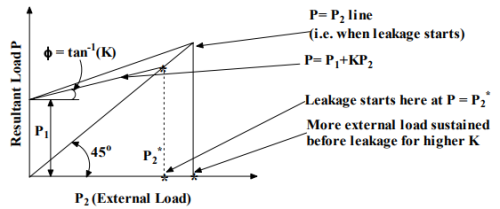4.4.3.1F – Force diagram for joint separation

Offer running on EduRev: Apply code STAYHOME200 to get INR 200 off on our premium plan EduRev Infinity!

## Machine Design

57 videos|71 docs|102 tests

,

,

,

,

,

,

,

,

,

,

,

,

,

,

,

,

,

,

,

,

,

;